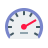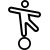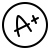# UPCOMING VERSION ANOP HINDI TYPING TUTOR 2.0 Preview

Exercise Name {{this.exeDataAll.test_name}}
{{exeLetter}}Click to {{ this.status == 'paused' ? 'Resume' : 'Start'}}
{{ Math.round(this.f_speed)}}WPMNet Speed Gross Speed {{ Math.round(this.f_g_speed)}}WPM## Formula Used:Gross Speed = (Total Character Typed / 5) / Time TakenNet Speed = Gross Speed - [(Wrong Character Typed / 5) / Time Taken]Backspace, Space, Shift Keypress, Skipped Words are not counted for calculation.

# ANOP TYPING TUTOR{{ this.exerciseData.length }} Total Words
{{ this.c_Word + 1 }} Current Word
{{ this.f_g_speed>0 ? Math.round(this.f_g_speed) : '0' }} Gross Speed WPM
{{ Math.round(this.f_speed) }} Net Speed WPM
{{ Math.round(this.accuracy) }} Accuracy %
{{ this.time }}
{{ this.no_r_Word }} Words Right
{{ this.no_w_Word }} Words Wrong
{{ this.no_s_Word }} Words Skip
{{ this.no_r_Letter }} Letters Right
{{ this.no_w_Letter }} Letters Wrong
Tutorial Name {{this.dataProp.tutorial_name}}Click to {{ !this.running && this.status == 'running' ? 'Resume' : 'Start'}}
{{ Math.round(this.f_speed)}}WPMNet Speed Gross Speed {{ Math.round(this.f_g_speed)}}WPM## Formula Used:Gross Speed = (Total Character Typed / 5) / Time TakenNet Speed = Gross Speed - [(Wrong Character Typed / 5) / Time Taken]Backspace, Space, Shift Keypress, Skipped Words are not counted for calculation.
{{systemWord}} {{userWord}}
{{ this.var_lf_1 ? this.upCommingKey : "^" }} {{ this.var_lf_2 ? this.upCommingKey : "^" }} {{ this.var_lf_3 ? this.upCommingKey : "^" }} {{ this.var_lf_4 ? this.upCommingKey : "^" }} {{ this.var_rf_1 ? this.upCommingKey : "^" }} {{ this.var_rf_2 ? this.upCommingKey : "^" }} {{ this.var_rf_3 ? this.upCommingKey: "^" }} {{ this.var_rf_4 ? this.upCommingKey : "^" }}~
`
! !
1
@ /
2
# रु
3
\$ +
4
% :
5
^
6
& -
7
*
8
( ;
9
) द्ध
0
_ ऋ़
. -
+
त्र =
Backspace
Tab
Q
q
W
w
E म्
e
R त्
r
T ज्
t
Y ल्
y
U न्
u
I प्
i
O व्
o
P च्
p
{ क्ष्
ख् [
} द्व
, ]
| द्य
? \
Caps
Lock
A
a
S
s
D क्
d
F थ्
f ि
G
g
H भ्
h
J श्र
j
K ज्ञ
k
L स्
l
: रू
;
" ष्
श् '
Enter
Shift
Z Z
z z
X ग्
x
C ब्
c
V
v
B
b
N
n
M
m
<
,
>
ण् .
? घ्
ध् /
Shift
Ctrl
Win
Alt
Space
Alt
Win
Ctrl

# ANOP TYPING TUTOR{{ this.tuteData.length }} Total Words
{{ this.tuteCursur + 1 }} Current Word
{{ this.f_g_speed>0 ? Math.round(this.f_g_speed) : '0' }} Gross Speed WPM
{{ Math.round(this.f_speed) }} Net Speed WPM
{{ Math.round(this.accuracy) }} Accuracy %
{{ this.time }}
{{ this.no_c_right }} Letters Right
{{ this.no_c_wrong }} Letters Wrong